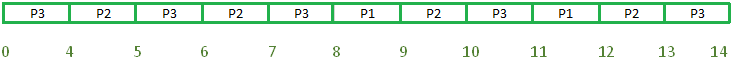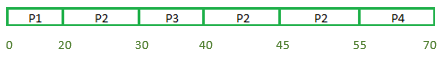# Difference between SRJF and LRJF CPU scheduling algorithms

• Difficulty Level : Easy
• Last Updated : 28 Dec, 2021

1. Shortest remaining job first (SRJF) :
Shortest remaining job first also called the shortest remaining time first is the preemptive version of the shortest job first scheduling algorithm.
In the shortest remaining job first, the process with the smallest runtime to complete (i.e remaining time) is scheduled to run next, In SRJF, a running process will be preempted if a new process arrives in the CPU scheduler which requires less burst time for execution.

2. Longest remaining job first (LRJF) :
Longest remaining job first also called longest remaining time first is exactly opposite of SRJF. In this type of CPU scheduling, the process which has the longest processing time is scheduled to run next and a running process will be preempted if a new process with longer burst time enters the queue.

Difference table :

Let’s solve one problem for each:
LJFT :

Longest remaining job first:
Gantt chart:Therefore the final table will be:
LJFT :

```Turn around time = Completion time - Arrival time

Average turn around time,
= [(12-0) + (13-0) + (14-0)]/3
= (12 + 13 + 14)/3
= 13

Waiting time = Turn around time - Burst time

Average waiting time,
= [(12-2) + (13-4) + (14-8)]/3
= (10 + 9 + 6)/3
= 8.34 ```

Problem two:

LJFT

LJFT

Shortest remaining time first:
Gantt chart:Therefore the final table will be:
SRFT

```Turn around time = Completion time - Arrival time

Average turn around time,
= [(20-0) + (55-15) + (40-10) + (70-45)]/4
= (20 + 40 + 30 + 25)/4
= 28.75

Waiting time = Turn around time - Burst time

Average waiting time,
= [(20-20) + (40-25) + (10-10) + (25-15)] / 4
= (0 + 15 + 0 + 10) / 4
= 6.25 ```

My Personal Notes arrow_drop_up